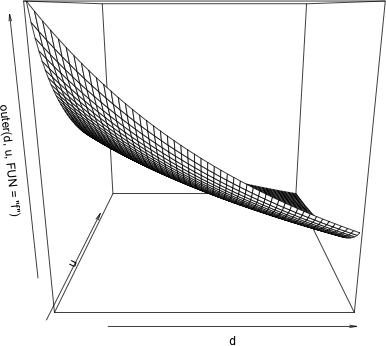# 懒人如何判断二元函数的单调性

### 谢益辉 2005-07-28

$$f(d,u)={1/(d-u)-(\log(d/u))*u/(d-u)^2},\quad d\in(0.5,1),,u\in(0,0.5)$$

:) d_seq(0.51,1,0.01)
:) u_seq(0,0.49,0.01)
:) f_function(d,u)
^^ {1/(d-u)-(log(d/u))*u/(d-u)^2}
:) d_seq(0.51,1,0.01)
:) u_seq(0,0.49,0.01)
:) persp(d,u,outer(d,u,FUN="f"),"d","u","f")**Outer**

**DESCRIPTION**

Performs an outer product operation given two arrays (or vectors) and, optionally, a function.

**USAGE**

outer(X, Y, FUN="*", ...)

X %o% Y #operator form

**REQUIRED ARGUMENTS**

X,Y　　　first and second arguments to the function FUN. Missing values (NAs) are allowed if FUN accepts them.

**OPTIONAL ARGUMENTS**

FUN　　　an S-PLUS function that takes at least two vectors as arguments and returns a single value. This may also be a character string giving the name of such a function.
...　　　other arguments to FUN, if needed.  The names of the arguments, if any, should be those meaningful to FUN.

**VALUE**

array, whose dimension vector is the concatenation of the dimension vectors of X and Y, and such that FUN(X[i,j,k,...], Y[a,b,c,...]) is the value of the [i,j,k,...,a,b,c,...] element. (Vectors are considered to be one-dimensional arrays.)

**DETAILS**

outer forms two arrays corresponding to the data in X and Y, each of which has a dim attribute formed by concatenating the dim attributes of X and Y. It then calls FUN just once with these two arrays as arguments. Therefore, FUN should be a function that operates elementwise on vectors or arrays and expects (at least) two arguments.

crossprod, Matrix-product.

**EXAMPLES**

z <- x %o% y   # The outer product array

# dim(z) == c(dim(as.array(x)), dim(as.array(y)))

# simulate a two-way table

outer(c(3.1, 4.5, 2.8, 5.2), c(2.7, 3.1, 2.8),"+") + matrix(rnorm(12), nrow=4)

z <- outer(months, years, paste) # All month, year combinations pasted.

**Persp**

**DESCRIPTION**

Creates a perspective plot given a matrix that represents heights on an evenly spaced grid. Axes and/or a box may be placed on the plot.

**USAGE**

persp(x, y, z, xlab = "X", ylab = "Y", zlab = "Z", axes = T,
box = T, eye = <<SEE below="" />>, zlim = <<SEE below="" />>, ar = 1)

**REQUIRED ARGUMENTS**

x　　　vector containing x coordinates of the grid over  which  z is evaluated. The values should be in sorted order; missing values are not accepted.
y　　　vector of grid y coordinates. The values should be in sorted order; missing values are not accepted.
z　　　matrix of size length(x) by length(y) giving  the  surface height  at grid points, i.e., z[i,j] is evaluated at x[i], y[j].  The rows of z are indexed by x, and the columns  by y.  Missing values (NAs) are allowed, but should generally not occur in the convex hull of the non-missing values.

The first argument may be a list with components x, y, and z. In particular, the result of interp is suitable.

If the first argument is a matrix, it is assumed to be the z matrix and vectors x and y are generated (x is 1:nrow(z), y is 1:ncol(z)).   The result of
predict together with expand.grid is suitable as an argument to persp.

**OPTIONAL ARGUMENTS**

xlab　　　character label for the x axis.
ylab　　　character label for the y axis.
zlab　　　character label for the z axis.
eye　　　vector giving the x,y,z coordinates for the viewpoint. The default is c(-6, -8, 5) times the range of the x, y, and z values.
axes　　　logical flag: should axes be drawn around the surface?
box　　　logical flag: should a box be drawn around the surface?

zlim　　　vector of the desired minimum and maximum z axis values.  The default is the range(z, na.rm=T).  This range will be expanded to round numbers.  The values in the input matrix z will be truncated to the z axis limits.
ar　　　aspect ratio for plotting the x,y grid, i.e., (xmax-xmin)/(ymax-ymin).

Graphical parameters may also be supplied as arguments to this function (see par).

**NOTE**

persp assumes that the grid vectors x and y are increasing and evenly spaced.  If this is not true, suppress the axes with axes=F or use par commands to customize the axes.

**VALUE**

invisible list containing information about what was plotted.

rotation　　　integer flag between 1 and 4 describing the point of view based on which corner of the xy plane appears closest to the viewer.
proj　　　2 by 2 by 2 array describing the projection of vectors that span the data.
rangex　　　vector giving the min and max values for x.
rangey　　　vector giving the min and max values for y.
rangez　　　vector giving the min and max values for z.

**SIDE EFFECTS**

a perspective plot is created on the current graphics device.

**DETAILS**

Use persp.setup to change the color, line width and line type of the perspective lines.  Use the perspp function to add information to a perspective plot.

**BUGS**

For some plots (including the case where mfrow or mfcol is bigger than c(2,2)) parts of the axes are cut off.  You can solve this problem either by putting up fewer figures on the page, changing the perspective angle with the eye argument, or by expanding the margin in which the cut off occurs.

Lines with an endpoint outside the plot may not be drawn.

contour, expand.grid, image, interp, outer, par, persp.setup, perspp, predict.

**EXAMPLES**

#Example 1, a polynomial

poly1 <-function(x, y){x^2 + x * y + y^2}
y <- x <- seq(-25, 25, length = 50)
persp(x,y, outer(x,y, FUN =  poly1))

#Example 2, data at geographic points
i <- interp(ozone.xy$x,ozone.xy$y,ozone.median)
persp(i, xlab = "Longitude", ylab = "Latitude", zlab = "Ozone")
title(main = "Median Ozone Concentrations in the North East")

#Example 3, first argument is a matrix
persp(switzerland)

#Example 4, a smooth surface
int.ak <- interp(akima.x, akima.y, akima.z)
r.ak <- sapply(int.ak, function(x) diff(range(x,na.rm = T)))

par(mfrow = c(2,2), oma = c(0,0,5,0))
persp(int.ak)
persp(int.ak, eye = c(6,-8,5)*r.ak)
persp(int.ak, eye = c(6,8,5)*r.ak)
persp(int.ak, eye = c(-6,8,5)*r.ak)
mtext(outer = T, side = 3, line = 2, "WAVEFORM DISTORTION", cex = 1.5)

par(mfrow = c(1,1))
persp(int.ak, eye = c(6,8,5)*r.ak)
title(main = "WAVEFORM DISTORTION")